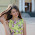## Pages

### Python - Variable Types

Assigning Values to Variables:

In python, variables are automatically declared when we assign a value to a variable. We use equal sign (=) to assign value to variable. For example:
a = 100                  #integer assignment
name = "linux"       # floating point
num = 244.4412   # string

Multiple Assignment:

Assigning single value to many variables:
a = b = c = 1
Assigning multiple objects to multiple variables:
a , b , c = 30, 5.54, "hello"
here  a= 30 , b = 5.54, c = hello

Standard data types:

Five standard data types python are numbers, strings, list, tuple, dictionary

Python Numbers:

These store numeric values.They supports int, long, float, complex.

Python Strings:

Strings in Python are identified as a contiguous set of characters in between quotation marks. We can use either pairs of single or double quotes. For example:
a = 'HELLO'
or
a="HELLO"
now,
print a will print 'H'
print a[1:3] will print 'ELL'
print a[2:] will print 'LLO'
print a*2 will print 'HELLOHELLO' ie it print the string two times.
print a + ' WORLD' will print 'HELLO WORLD' ie concatenation of string

Python List:

Lists are the most versatile of Python's compound data types. A list contains items separated by commas and enclosed within square brackets ([]).
To some extent, lists are similar to arrays in C. One difference between them is that all the items belonging to a list can be of different data type.
The values stored in a list can be accessed using the slice operator ( [ ] and [ : ] ) with indexes starting at 0 in the beginning of the list and working their way to end-1.
The plus ( + ) sign is the list concatenation operator, and the asterisk ( * ) is the repetition operator. For example:

list = ['abcdef', 555, 434.53, 'vinod', 43, 54+5j ]

It is easy to access list elements. Accessing list is similar to that of accessing string characters ie in a list each element could be imagined as characters in a string.
print list will print 'abcdef'
and rest is similar to that of strings discussed above. We can update list. (read and write allowed)

Python Tuples:

A tuple is another sequence data type that is similar to the list.
differences between tuple and list are:
• Tuples are enclosed within parenthesis but lists with square brackets.
• Tuples are READ ONLY but lists are not.
So we cannot update tuples but we can update list.

Python Dictionary:

Python dictionary consist of key-value pairs. Keys can be almost any Python type, but are usually numbers or strings. Values, on the other hand, can be any arbitrary Python object. Dictionaries are enclosed by curly braces ( { } ) and values can be assigned and accessed using square braces ( [] ).

For example:
(in python interactive interpreter)

root@home-pc:~# python
Python 2.5.2 (r252:60911, Oct  5 2008, 19:24:49)
[GCC 4.3.2] on linux2
>>> dict = {}
>>> dict['name'] = 'vinod'
>>> dict['age'] = 23
>>> dict['dept'] =' electronics'
>>> dict
{'dept': ' electronics', 'age': 23, 'name': 'vinod'}
>>> dict.keys()
['dept', 'age', 'name']
>>> dict.values()
[' electronics', 23, 'vinod']
>>>

#### 1 comment :

1.Very Well-Written Content. I found this blog unique and what i have been looking for, many thanks. Happy Independence Day Quotes For Friends in Marathi 2018